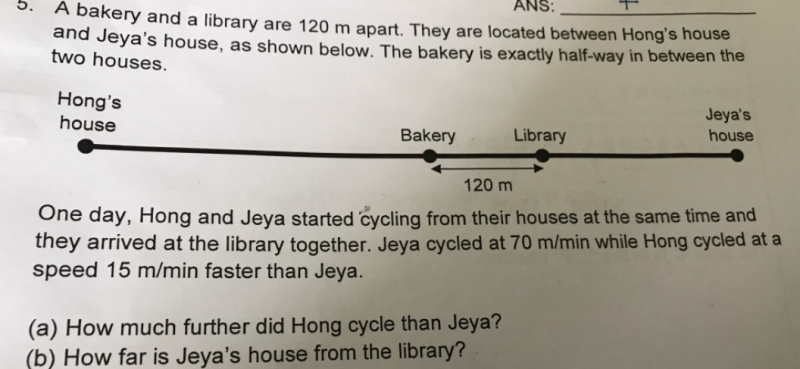# QuestionAppreciate any help

The working is correct. However, as b) of the question asks : “How far is Jeya’s, not Jaya’s, house from the library?”, it should be 14 units = 1120 km instead of  ’17 units = 1360km’, which is the distance between Hong’s house and the library.

My solution is as follows

(a)

120 x 2 = 240

(b)
distance between Hong’s house or Jeya’s house from the bakery : 1u
(1u + 120)/(70 + 15) ——- (1u – 120)/70
70u + 8400 ——- 85u – 10200
1u ——- (8400 + 10200)/(85 – 70) = 1240
1240 – 120 = 1120

Ans : (a) 240 m; (b) 1120 m.

thanks.

1 Reply 0 Likes

My pleasure.

0 Replies 0 Likes

Appreciate any one to see if this working is correct

Hong    :     Jaya    : diff

Actual speed               85    :       70   :  15

Ratio speed                 17    :       14     :      3

Ratio distance              17    :       14      :       3

A. U +120-(u-120)=240

3 units = 240

1 unit = 80

A)17 units = 1360km

1 Reply 0 Likes

The working is correct. However, as b) of the question asks : “How far is Jeya’s, not Jaya’s, house from the library?”, it should be 14 units = 1120 km instead of  ’17 units = 1360km’, which is the distance between Hong’s house and the library.

My solution is as follows

(a)

120 x 2 = 240

(b)
distance between Hong’s house or Jeya’s house from the bakery : 1u
(1u + 120)/(70 + 15) ——- (1u – 120)/70
70u + 8400 ——- 85u – 10200
1u ——- (8400 + 10200)/(85 – 70) = 1240
1240 – 120 = 1120

Ans : (a) 240 m; (b) 1120 m.